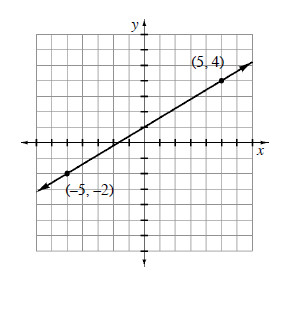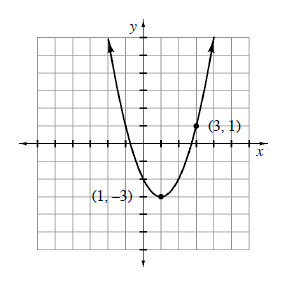### Home > CALC > Chapter 1 > Lesson 1.2.4 > Problem1-68

1-68.

Find a possible function for each of the following graphs. Verify your equation on your graphing calculator. .

1.You could use $y = mx+b$ form. The slope and $y$-intercept are easy to see.

Or you could use point-slope form of a line.

1.Notice that this is a translation of $y = x^2$.